### 北京奔驰 奔驰GLA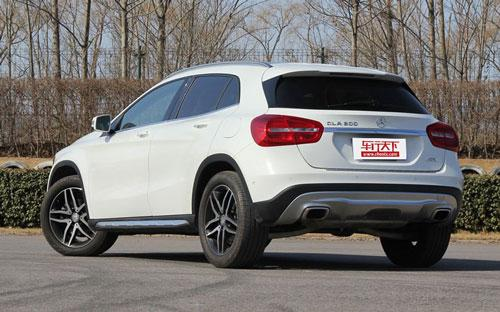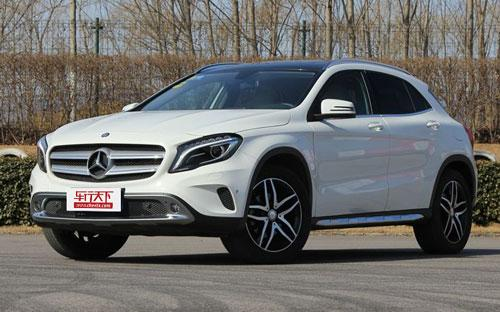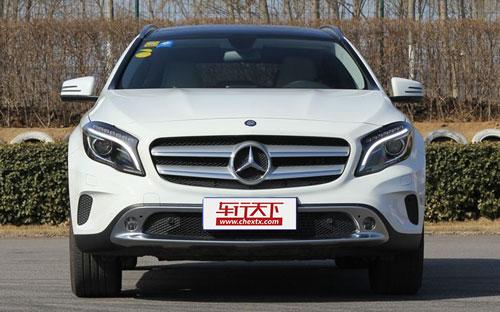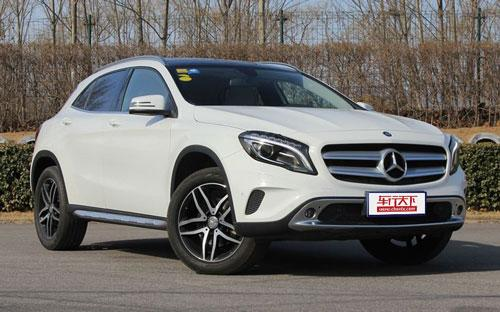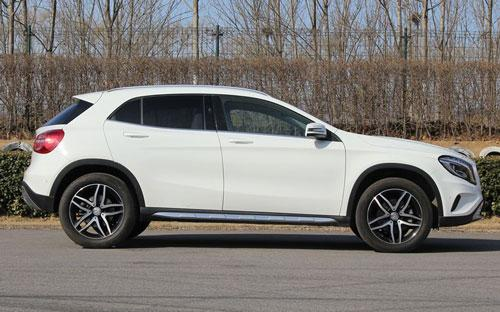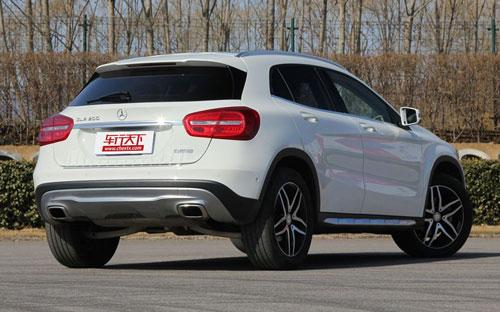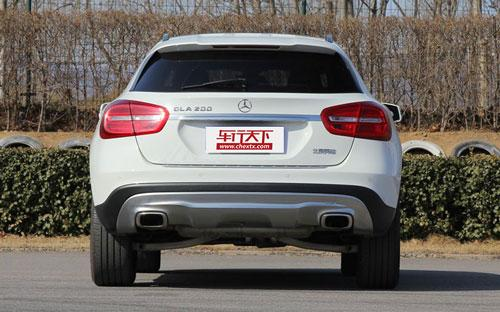7 种颜色可选2016款最低售价：26.98 万元起

4431(mm)1804(mm)1532(mm)##### 配置亮点：
• 胎压监测装置

• ISOFIX儿童座椅接口

• 车身稳定控制(ESC/ESP/DSC等)

• 电动天窗

• 定速巡航

• 后倒车雷达

• 真皮座椅

• GPS导航系统

• 氙气大灯

• 后视镜加热

• 提交
2016款 GLA 220 4MATIC 豪华型 (144张)
• 2016款 GLA 220 4MATIC 豪华型 (144张)
• 2016款 GLA 200 动感型 (139张)
• 2016款 GLA 200 时尚型 (138张)
• 2016款 GLA 220 4MATIC 时尚型 (146张)
• 北京奔驰 奔驰GLA 绕车实拍• 北京奔驰 奔驰GLA 在售车型

排量 车型
5
-
-
-
-
-
5
-
-
-
-
-
5
-
-
-
-
-
6
-
-
-
-
6
-
-
-
-
6
-
-
-
-
5
-
-
-
-
-
6
-
-
-
-
6
-
-
-
-
6
-
-
-
-
厂商指导价 本地最低报价 购车工具
1.6L
GLA 200 动感型 7挡双离合

26.98万
26.98万

2.0L
GLA 200 动感型 7挡双离合

26.98万
26.98万

1.6T
GLA 200 时尚型 7挡双离合

28.98万
28.98万

GLA 220 4MATIC 时尚型 7挡双离合

31.98万
GLA 220 4MATIC 豪华型 7挡双离合

34.98万
GLA 260 4MATIC 运动型 7挡双离合

39.80万
39.80万

2.0T
GLA 200 时尚型 7挡双离合

28.98万
28.98万

GLA 220 4MATIC 时尚型 7挡双离合

31.98万
GLA 220 4MATIC 豪华型 7挡双离合

34.98万
GLA 260 4MATIC 运动型 7挡双离合

39.80万
39.80万

北京奔驰 奔驰GLA 经销商

查看更多 >>

### 北京奔驰 奔驰GLA 动力加速

奔驰GLA 0-100公里加速时间分布在 7.2-9.2秒 属于 运动级

动力级别 加速时间 车型
民用级(1款)
9.2s
GLA 200 时尚型
运动级(2款)
7.9s
GLA 220 4MATIC 时尚型GLA 220 4MATIC 豪华型
7.2s
GLA 260 4MATIC 运动型

北京奔驰 奔驰GLA 视频

北京奔驰 奔驰GLA 新闻资讯

# 北京奔驰新款GLA上市 售价26.98-39.80万

国产新车 超过8393次关注

据奔驰官方获悉，国产奔驰GLA推出2016款车型，新车在外观方面并没有太大变化，搭载1.6T和2.0T两款发动机，共计5款车型，售价不变，仍为26.98-39.80万。

# 国产奔驰GLA 260性能全方位测试

评测 超过12813次关注

近年来奔驰迅速开辟紧凑型车战线，新A级、CLA级、GLA级都是奔驰用来主攻年轻市场的产品，其中GLA的封顶价格最高，达到了39.8万元。

# 输出122马力 曝北京奔驰GLA 180实车照

新闻 超过9051次关注

日前，有国内媒体捕获到了一组北京奔驰GLA180的实车照，新车将搭载的是一台低功率1.6T涡轮增压发动机，我们预计这款车将于年内投放市场。

# 售26.98万起 国产奔驰GLA亮相上海车展

新闻 超过8004次关注

在刚刚开幕的2015上海车展上，国产奔驰GLA正式上市。新车共提供五款车型选择，售价区间为26.98-39.80万。

# 买车不用多花钱 豪华品牌紧凑型SUV推荐

汽车导购 超过17173次关注

在奔驰GLA级上市之后，豪华品牌紧凑型SUV差不多就到齐了，它们的入门车型已经下探到25-30万元的区间，所以不少打算购买这个价位合资SUV的朋友都将目光转投在了它们身上。

猜你喜欢

﻿
• 快速找车
• 选择品牌
• 选择品牌
• A  奥迪
• A  阿斯顿·马丁
• A  阿尔法·罗密欧
• B  宝沃
• B  布加迪
• B  巴博斯
• B  保时捷
• B  宾利
• B  奔驰
• B  宝马
• B  本田
• B  别克
• B  标致
• B  比亚迪
• B  宝骏
• B  北汽制造
• B  北汽新能源
• B  北汽幻速
• B  北汽威旺
• B  北京汽车
• B  奔腾
• B  北汽绅宝
• C  长安
• C  长安商用
• C  长城
• C  昌河
• D  大众
• D  道奇
• D  DS
• D  东南
• D  东风风神
• D  东风风行
• D  东风小康
• D  东风风度
• D  东风
• F  福特
• F  丰田
• F  菲亚特
• F  法拉利
• F  福田
• F  福迪
• F  福汽启腾
• G  观致
• G  广汽传祺
• G  广汽吉奥
• G  GMC
• H  红旗
• H  汉腾汽车
• H  哈弗
• H  哈飞
• H  海格
• H  海马
• H  华颂
• H  黄海
• H  华泰
• H  恒天
• J  吉利汽车
• J  捷豹
• J  Jeep
• J  江淮
• J  江铃
• J  金杯
• J  九龙
• J  金旅
• K  凯翼
• K  凯迪拉克
• K  克莱斯勒
• K  科尼塞克
• K  卡威
• K  开瑞
• L  路虎
• L  林肯
• L  劳斯莱斯
• L  兰博基尼
• L  雷克萨斯
• L  铃木
• L  雷诺
• L  理念
• L  力帆
• L  莲花汽车
• L  猎豹
• L  路特斯
• L  陆风
• M  马自达
• M  MG
• M  MINI
• M  玛莎拉蒂
• M  摩根
• M  迈凯轮
• N  纳智捷
• O  欧宝
• O  讴歌
• O  欧朗
• Q  奇瑞
• Q  起亚
• Q  启辰
• R  日产
• R  荣威
• R  瑞麒
• S  三菱
• S  斯威汽车
• S  萨博
• S  smart
• S  斯柯达
• S  斯巴鲁
• S  思铭
• S  双龙
• S  上汽大通
• S  双环
• T  特斯拉
• T  腾势
• W  沃尔沃
• W  五菱汽车
• W  五十铃
• W  威兹曼
• W  威麟
• X  现代
• X  雪佛兰
• X  雪铁龙
• X  西雅特
• Y  一汽
• Y  英菲尼迪
• Y  英致
• Y  依维柯
• Y  野马汽车
• Y  永源
• Z  众泰
• Z  中华
• Z  中兴
• Z  知豆
• 选择车系
• 选择车系
• 车型对比
• 选择品牌
• 选择品牌
• A  奥迪
• A  阿斯顿·马丁
• A  阿尔法·罗密欧
• B  宝沃
• B  布加迪
• B  巴博斯
• B  保时捷
• B  宾利
• B  奔驰
• B  宝马
• B  本田
• B  别克
• B  标致
• B  比亚迪
• B  宝骏
• B  北汽制造
• B  北汽新能源
• B  北汽幻速
• B  北汽威旺
• B  北京汽车
• B  奔腾
• B  北汽绅宝
• C  长安
• C  长安商用
• C  长城
• C  昌河
• D  大众
• D  道奇
• D  DS
• D  东南
• D  东风风神
• D  东风风行
• D  东风小康
• D  东风风度
• D  东风
• F  福特
• F  丰田
• F  菲亚特
• F  法拉利
• F  福田
• F  福迪
• F  福汽启腾
• G  观致
• G  广汽传祺
• G  广汽吉奥
• G  GMC
• H  红旗
• H  汉腾汽车
• H  哈弗
• H  哈飞
• H  海格
• H  海马
• H  华颂
• H  黄海
• H  华泰
• H  恒天
• J  吉利汽车
• J  捷豹
• J  Jeep
• J  江淮
• J  江铃
• J  金杯
• J  九龙
• J  金旅
• K  凯翼
• K  凯迪拉克
• K  克莱斯勒
• K  科尼塞克
• K  卡威
• K  开瑞
• L  路虎
• L  林肯
• L  劳斯莱斯
• L  兰博基尼
• L  雷克萨斯
• L  铃木
• L  雷诺
• L  理念
• L  力帆
• L  莲花汽车
• L  猎豹
• L  路特斯
• L  陆风
• M  马自达
• M  MG
• M  MINI
• M  玛莎拉蒂
• M  摩根
• M  迈凯轮
• N  纳智捷
• O  欧宝
• O  讴歌
• O  欧朗
• Q  奇瑞
• Q  起亚
• Q  启辰
• R  日产
• R  荣威
• R  瑞麒
• S  三菱
• S  斯威汽车
• S  萨博
• S  smart
• S  斯柯达
• S  斯巴鲁
• S  思铭
• S  双龙
• S  上汽大通
• S  双环
• T  特斯拉
• T  腾势
• W  沃尔沃
• W  五菱汽车
• W  五十铃
• W  威兹曼
• W  威麟
• X  现代
• X  雪佛兰
• X  雪铁龙
• X  西雅特
• Y  一汽
• Y  英菲尼迪
• Y  英致
• Y  依维柯
• Y  野马汽车
• Y  永源
• Z  众泰
• Z  中华
• Z  中兴
• Z  知豆
• 选择车系
• 选择车系
• 选择车型
• 选择车型
• 意见反馈